# Abstract

A dimmer is an electronic device that controls alternate voltage applied to a lamp through delivering a selected portion of the mains sinusoid. Engineer, designing a dimmer needs to estimate how big this portion should be to get a desired luminance level. This article uses a model of incandescent lamp, tungsten resistivity, and human eye spectral efficiency to derive dependency of produced luminous flux over the voltage, gives a simple analytical function that describes this dependency with good accuracy (±2% comparing to the model).

# Structured Abstract

 Purpose To discover a dependency of luminous flux produced by an incandescent lamp on the applied voltage Methodology/Approach Numerical analysis on a theoretical model of an incandescent lamp Findings Analytical function for approximate estimating of luminous flux for the applied voltage with ±2% accuracy Research limitations/implications Model does not encounter thermal conduction and convection in the lamp. Practical implications (if applicable) Provided function has some degree of dependency on the lamp’s design (namely, nominal filament temperature)

# Definitions

Process of controlling some output value (such as voltage `U` or luminous flux `L`) we will denote as a control function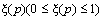, defined on the control parameter: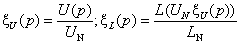(1)

Each ofmonotonously increase on the defined range. Control functions defined on a different (other than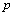) argument we will denote in this article as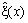.

Commonly used are linear () and logarithmic (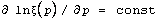) control functions. To implement a desired control function, we needs to know what is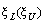dependency.
The following chapters give an attempt to deriveusing a model suggested in (Agraval 1996).

# Incandescent Lamp Model

An incandescent lamp is characterized with few nominal values: PN – power consumption, UN – nominal voltage, LN – nominal luminous flux, produced by the lamp at nominal voltage. The flux is produced by filament, incandesced to nominal working temperature TN.
When lower voltage U<UN is applied, operating temperature T of filament is lower TN and therefore it produces less luminosity L.

## Filament Resistance

Power, consumed by a lamp, is expressed with Ohm low:(2)

where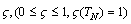defines dependency of filament resistance over the temperature relatively to its nominal resistance RN, which can be evaluated via PN and UN:(3)

Dependency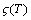for tungsten is not linear in the working range of temperatures (1000-2500K). For this model we will use polynomial approximation of tungsten resistance ρ, [Harang 2003]:(4)can be expressed via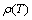as the following:(5)

## Filament Temperature

During operation, filament radiates electromagnetic energy and dissipates heat via conduction and convection. To estimate radiation, filament is modeled [Agraval 1996] as a simple non-ideal blackbody that obeys Plank’s radiation law: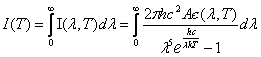(6)

where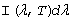is power radiated between wavelength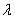and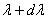,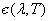– tungsten emittance, and A is filament area. The total power emitted over all wavelengths is: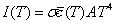(7)

where `σ` is the Stefan-Boltzman constant andis average emittance over all wavelengths, which is approximated as a second order polynomial (Harang 2003):(8)

In a steady-state operation, power applied to the lamp is in balance with power radiated and dissipated to outside ambient. Dissipation has two constituents – conduction and convection. Agraval in [Agraval 1996] has suggested a reasonable way of accounting dissipation as a factor of input power: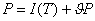(9)

Solving (8) for P: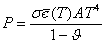(10)

Substituting left side of (10) with right side of (2):(11)

Using (11), we may derive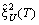: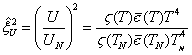(12)

Figure 1 illustrates dependency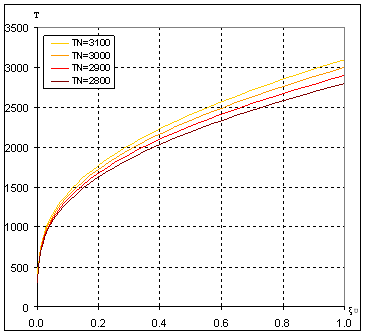Figure 1. Dependency of T on fraction of applied voltage ξU for different TN

## Luminance

Not all power radiated by filament has its effect on luminous flux. Some of the energy is absorbed by bulb glass and dissipated as heat. Although, this absorption depends on λ, we will simplify this absorption to a constant factor η<1.

Major part of the emitted energy is not visible to human eye. This is described as spectral efficiency function S(λ), approximated as the following [Agraval 1996]:(13)

Thus, total luminous flux produced by a lamp can be defined as: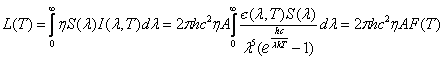(14)

where(15)

Thereby, control function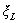can be expressed as a function of T: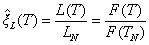(16)

For working range of temperatures and visible area, emissivity є(λ,T) can be approximated as the following [Larrabee 1957]:(17)

where T is in °K and λ in nm. Substituting (17) in (16) and integrating numerically (16) we get dependency, shown on Figure 2: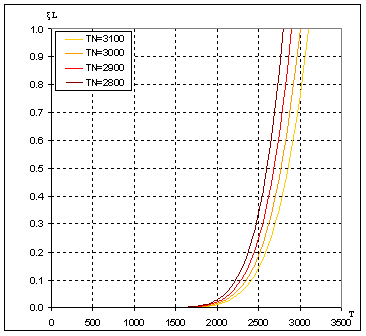Figure 2. Dependency ξL(T) for different TN

# Solving Luminance vs. Voltage

Using T as a parametric variable, we may numerically compute (11), (15) and graphically solve dependency ξLU), as shown on Figure 3: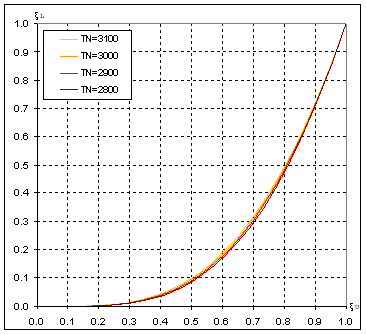Figure 3. Dependency ξL(ξU) for different TN

As Figure 3 indicates, ξL(ξU) is affected by a design factor – nominal temperature TN.

# Approximation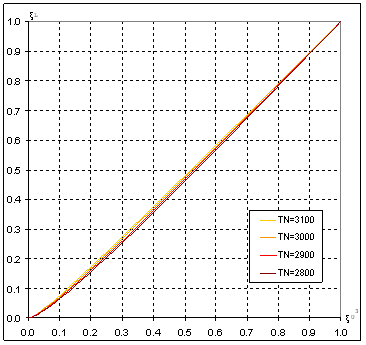Figure 4. Dependency ξLU3)

As it appears (see Figure 4), dependency ξL(ξU3) looks quite linear for ξU3>0.2 and has some higher-order dependency for ξU3<0.2. Therefore we will try approximating ξL(ξU) as two polynoms: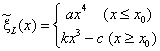(18)

Value ξLat `x=1` is equal to `1` by nature of ξ, therefore `c` can be expressed via `k`: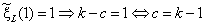(19)

To solve `a` and `x`0 we will require continuality ofand its derived function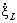, this gives us two equations with three unknowns:(20)

Solving (20) for `a` and `x`0 we get them expressed via `k`:(21)

Thereby,depends on a single constant k, which is then wiggled around to find a value giving lowest RMS deviation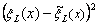. Table 1 lists selected values for few most common nominal temperatures TN, Figure 5 illustrates this dependency, and Figure 6 shows modeled curves and values, approximated with (20).

Table 1. k values for some TN

 TN k 2800 1.067 2900 1.054 3000 1.043 3100 1.034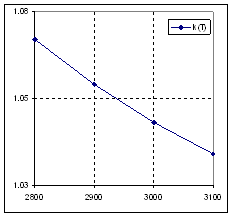Figure 5. k variations over TN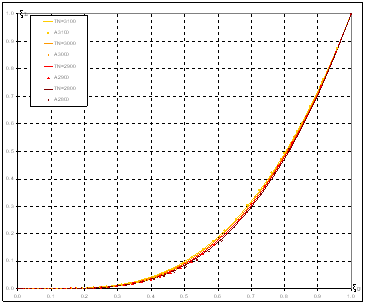Figure 6. Modeled curves and approximated values (marks)

Since designer of a dimmer may not exactly know nominal temperature of the dimmed lamp, `k`can be selected for an average nominal temperature. Suggested value `k=1.05` gives ±2% error (against the model) for TN in range 2800-3100 °K. For this `k`, equation (20) becomes practically concrete:(22)

# Sine Wave Dimmer

A sine wave dimmer implemented with pulse-width modulation controls applied voltage with a cycle duty, which we will denote as control function ξt. Modulation frequency is usually chosen much higher than mains frequency. Therefore, RMS of output voltage is proportional to cycle duty, and control function ξU as simple as:(23)

To make ξL(p), linear on parameter p, function ξt(p) should be defined as the following: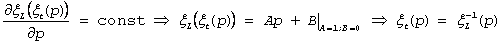(24)

Applying (24) to equation (22) we get(25)

# Phase Control Dimmer

When implementing an AC dimmer based on phase control technique, one needs to tabulate cycle duty values `ti` as a function of desired luminance level `pi`
Following the same approach, we introduce control function ξt: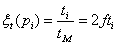(26)

where `t` is cycle duty (time when the switch is on) and `tM` is the mains half period, and `f` is the mains frequency. Average powerapplied to the lamp, can be evaluated as the following:(27)

where `UA` is voltage amplitude. Integrating (27) we can define ξP as the following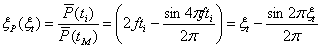(28)

Considering that, and substituting (28) to (22) we may estimate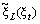.Figure 7. Dimmer control functions ξL and ξU

Applying approach used in (24) function ξt(p) is defined as following: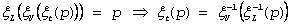(29)

Although, reverse function of (28) is not solvable analytically, it can be solved numerically.

# Conclusions

As a result of numerical modeling, the following approximation functions are suggested:

• Estimation of produced luminous flux over the voltage:

`L = L0 * ((U/U0 < 0.575) ? 1.369 * (U/U0) ^ 4 : (1.05 * (U/U0) ^ 3 - 0.05);`

• Tabulation of cycle duty over the control parameter p

`t = (p < 0.1496) ? (0.925 * (p) ^ -4) : (0.984 * (p + 0.05) ^ -3);`

# References

Agraval D.C., Leff H.S., Monon V.J. (1996)
"Efficiency and efficacy of incandescent lamps"
American Journal of Physics, May 1996, Volume 64, Issue 5, pp. 649-654
http://scitation.aip.org/getabs/servlet/GetabsServlet?prog=normal&id=AJPIAS000064000005000649000001&idtype=cvips&gifs=yes

Harang O., Kosch M. J. (2003) "Absolute Optical Calibration Using a Simple Tungsten
Bulb"
Sodankylä Geophysical Observatory Publications, 2003, pp. 92:121-123
http://spaceweb.oulu.fi/28AM/proc_papers/27_harang_et_al_calibration_with_tungsten_bulb.pdf

Larrabee R.D. (1957) "The spectral emissivity and optical properties of tungsten"
Research Laboratory Of Electronics, Massachusetts Institute of Technology
http://dspace.mit.edu/bitstream/1721.1/4755/1/RLE-TR-328-04734719.pdf